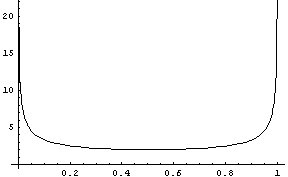## Friday, February 11, 2005

### Uninformative Priors

I came across an interesting article by Zhu and Lu talking about how non-informative priors can often be counter-intuitive. For instance suppose we are estimating parameter of a Bernoulli distribution. If we don't know anything, the most intuitive prior to use is uniform. However Jeffreys' "uninformative" prior doesn't look uniform at all:This prior seems to favour values of theta close to 0 or 1, so how can it be uninformative?

The intuition they provide is the following: think of Maximum Likelihood estimator as the most uninformative estimate of parameters. Then we can rank informativeness of priors by how closely the Bayesian Posterior Mean estimate comes to the maximum likelihood estimate.

If we observed k heads out of n tosses, we get following estimates:

1. MLE: k/n
2. PM using Jeffrey's prior (k+1/2)/(n+1)
3. Laplace smoothing (Uniform) (k+1)/(n+2)
4. PM using Beta(a,b) prior (k+a)/(n+a+b)

You can see that using this informal criteria for informativeness, Jeffreys' prior is more uninformative than Uniform, and Beta(0,0) (the limiting distribution) is the least informative of all, because then Posterior Mean estimate will coincide with the MLE.

Resources: